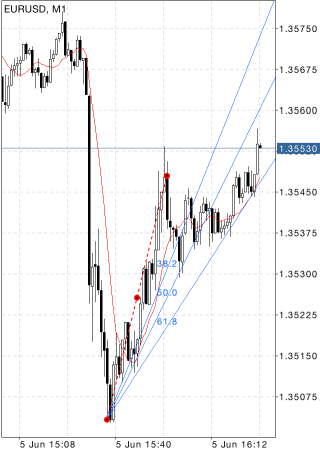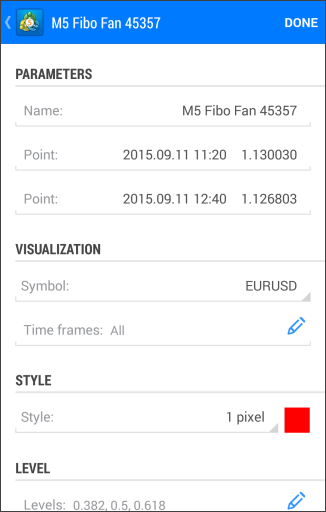MetaTrader 5 Android HelpChartsObjectsFibonacci ToolsFibonacci Fan

# Fibonacci Fan

Fibonacci Fan as a line instrument is built as follows: a trendline – for example from a trough to the opposing peak is drawn between two extreme points. Then, an "invisible" vertical line is automatically drawn through the second extreme point. After that, three trend lines intersecting this invisible vertical line at Fibonacci levels of 38.2, 50, and 61.8 percent are drawn from the first extreme point.

These lines are considered to represent support and resistance levels. For getting a more precise forecast, it is recommended to use other Fibonacci tools along with the Fan.

## Drawing

To draw Fibonacci Fan, select this object and set an initial point on the chart.After that drag your finger to draw a trendline of a desired length in the necessary direction. Additional parameters will be shown near the end point of the trendline: distance from the initial point along the time axis and distance from the initial point along the price axis, as well as the slope angle relative to a horizontal line drawn through the initial point at the scale 1:1.## Management

On the main trendline there are three points that can be dragged. The first and the last points are used for changing the length and direction of lines. The central point (moving point) is used for moving Fibonacci Fan without changing its dimensions and direction.

## Parameters

Fibonacci Fan has the following parameters:

• Point – coordinates of the initial point of the trend line (date/value of the price scale);
• Point – coordinates of the end point of the trend line (date/value of the price scale);

Common parameters of object are described in a separate section.